TWiki>Main Web>MatiasAlejandroBonaventura>TDAQSimulation>TDAQSimulationGroupCommunication>PhaseISimulation>PhaseIHybridSwitch (2016-08-26, MatiasAlejandroBonaventura)

# Hybrid Switch

## Idea

### Motivation

Qc => continous queue size
Qd => discrete queue size
Wc => continous flow (window size of TCP)

We identified the following drawbacks in the hybrid models:

1. The calculation of the Qc depends on the particular implementation of Wc (in this case a TCP sliding window affeced by AQM discards).
2. The Qc is calculated totally independent from the discrete flows. It assumes that the discrete flows dont affect and can be neglected.
1. Thus, if the calculation of Qc moves away from the discrete queue size, this is not percived by the model and might create errors
3. To implement Wc you need an equation estimating the discard probability and delay.
Thus, any implementation of Wc will be very particular to a specific setup (example: AQM routers or TCP). The idea is to remove these drawbacks and create an hybrid model that works with any implementation of Wc. Also discrete and continous flows should naturally interact and affect each other.

Also in the TCP-AQM model:

1. The hybrid queue implementation is very tight to AQM discards. The queue is set to infinite capacity and the AQM model implements discards based on probabilities which are very convenient for mixing discrete and continous representations. But if we want an hybrid queue without AQM there is no trivial way with this model (for example to handle discards).
2. If there is no discrete packets continous information does not get to the queue. For example if the queue gets filled only by the continous flow at the beggining and then a discrete flow joins.
An easy way to solve this would be sending packets with empty discrete part every now and then.
3. To solve the problem of discrete flows not giving feedback to the contious flows, the various samplers developed for this new queue could be used (periodSampler, rateSampler, QSSSampler(not implemented)).
Thus, we would like a model of an hybrid queue which is independent of how the Wc and discrete flows are implemented.
The main goal is to separate the concepts and implementation of 1) the switch and 2) the clients (senders) which can be either continuous or discrete. The goal is to provide an hybridSwitch model which works independent of any implementation of the clients (discrete, continuous or both simultaneously). This hybridSwitch model can be seen as a black blox receiving discrete and/or continuous flows, and applying adequate delays and discards. In the discrete world it has to apply delays and discards to individual packets (the model outputs discrete packets with a delay and some are discarded). In the continuous world is has to provide the following information to the models implementing Wc: delay(t) and discardProb(t) (maybe queueSize(t), but the delay should be enough)

New Hybrid switch concept
In green are the discrete components. In blue the continuous components.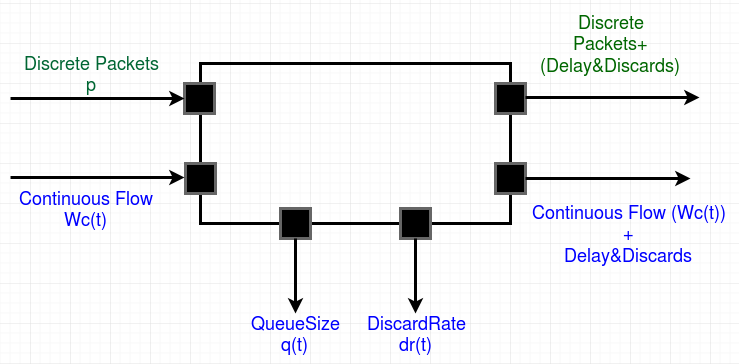The queueSize and discardRate are continuos components and sent through output ports because they are required as input to other continous models. They could also be discrete outputs for gathering metrics (the discrete queue size and actual discrete discards)), but because these values are not used by other models they are not outputs.
Also, the discrete packets and continous flow use different ports to ingress and egress of the model. Another design option is to have a single port for both flows and use hybrid packets instead (hybrid packets are discrete packets with a continuous component). Whatever design decition is taken, it should not change much the implementation as it is easy to join flows (the HybridFlow model) and to separate them.

To solve this problems, the idea is to move the queueSize calculation to the queue itself. For this purpose an hybrid switch (queue+server) is developed.
From the flow perspective, the queue+server functionality is basically to forward incoming packets after a delay. This delay depends on the size of the queue. Below is the idea on how to calculate these 2 values (queue size and delay). In last section how to calculate packet discards.

### Definitions for queueSize calculation

To solve this problems, the idea is to move the queueSize calculation to the queue itself. For this purpose an hybrid switch is developed with the following characteristics:

• Inspired in Towsley, Ohsaki and others, it is very streight forward to think of the queue size dynamics in the following way:(1)

whereas queue size shall never be lower than 0,is the link capacity (speed at which packets are dequeued), andis the incomming flow of packets (speed at which packets are queued).
This is a very trivial and intuitive way of thinking about a fluid queue.

• In our model C is a fixed parameter.
• W can be decomposed into continous and discrete flows:•can be represented by any equation, not only the TCP-AQM one. The hybrid queue would then become agnostic on the fluid flows implementation.
•is given by the flow of discrete packets. For example, the discrete flow can be sensed and its rate can be used (at any desired level of granularity). The hybrid queue is then agnostic of the incoming discrete flows.
• The transformation from discrete packets to a continous signal is key and a sensible aspect to this model.
To start with, we will implement it as a simple sampling over an intervalPeriod, outputing the rate as a constant continous signal. This is similar/equivalent to what it is done in Towsley two-pass model. But it would be good to study it more deeply (to understand implications of the intervalPeriod, analyse its theoretica correctitud, etc.
Later, we realized this intervalPeriodSampling was not acurate enought for queueSize calculation and we developed a rateSampler. The rateSampler sends an output everytime it receives a packet to increase the continous queueSize calculation.
Even more, it could be implemented in such a way that its output is not a constant, but rather a "QSS signal" (with 1st, 2d, derivatives.., sending signals when quantums are exceeded, etc).
The setting of the samplingRate will also greatly affect the execution performance (simulation times).
• The total queue sizecan then be calculated as:(2)

### Definitions for delay calculation

Once the total queue size is calculated it is trivial to calculate the delay that each incomming packet will experience to tranverse the link (queue+server).
The total delay for a new incomming packet can be calculated as:(3)

### Future ideas

To fully test and validate this model, some interesting tests:

• Try with model than a single discrete flow.
Once this is tested and validated, this implementation can be further used to:
• In the TCP-AQM model, the calculation of the Q_c can be replaced directly by the output of this model.
• The incoming continos flow (Wc) can be also set as an output of the model after applying is the calculated delay. It woudl be trivial using DQSS!!!
• This would be useful for interconection switches.
NOTE: after having developed this model it turned out to be "very contious": queueSize, delay and discards are calculated in the continous world and those values used for the discrete packets. The continous estimations can be as precise as desired (but probably affecting performance). If low precition is set, this approach might lose the precition of the discrete world (the key desired feature of hybrid models).

## Implementation

A coupled model was developed to implement the hybrid switch: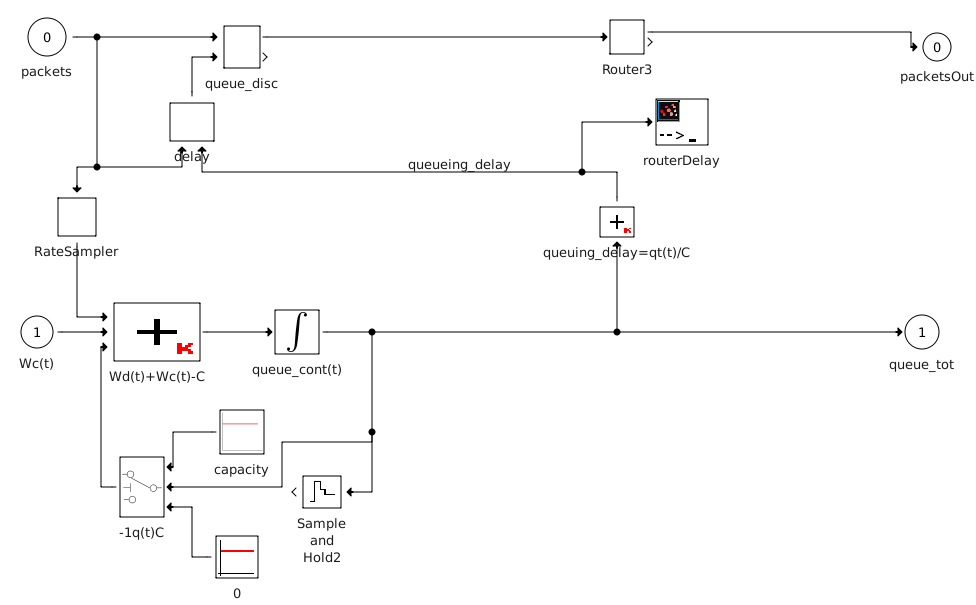All models are standard from the QSS library.
Except for the following onces which were newly developed:

• delay: This model controls the unqueing times of the queue applying a continuous queueing delay (received as parameter) to each packet. It would represent the server's processing time for the router. Calculates the propagation time based on the packet size, and adds the queueingDelay received as parameter. Packets are stored in the queue (not by this model), this model just signals the queue when to out them.
• RateSampler: Counts the number of arrived events and outputs its rate every samplePeriod.• Router3: This model is just for backwards compatibility. It just forwards received packets without any delay, but performs some packet manipulation (changes protocols). In the future it should be removed, maybe perform routing tasks.
The bottom area of the model is the contious part which implements theequation. It is used to implemente thesending at 1000 packets/sec. The capacity is C=1250. The discrete source sends packets of 500Bytes at maximum capacity (1250 packets/sec).

It is expected the queue to grow at 1000 packets per second. Same as the delay applied to discrete packets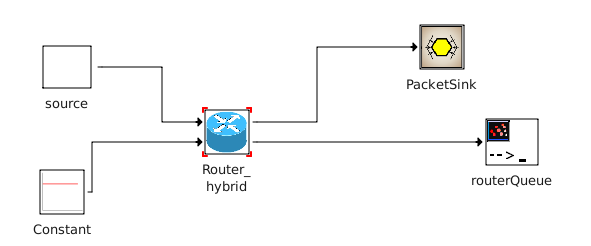Results (from left to right: 1)residence time for discrete packets, 2) queue size, and 3)queueing delay
As expected the queue grew 1000 packets after 1s. The delay and packetLatency grows accordingly.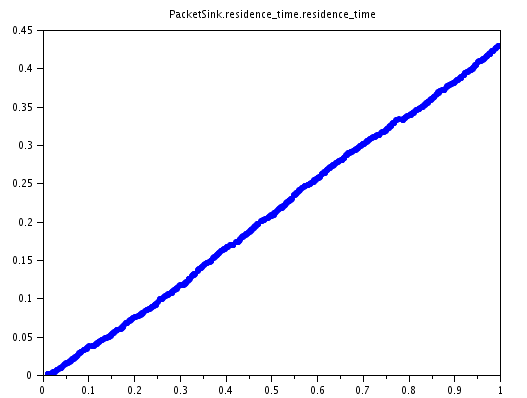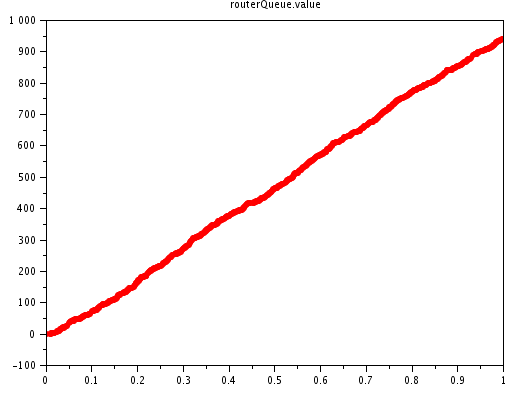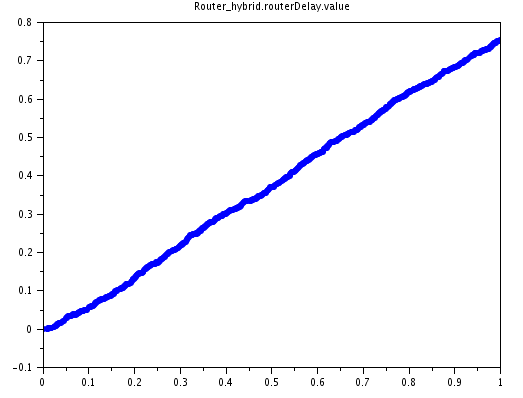### Testing low queueSize boundary (never lower than 0)

In the current implementation the -1q(t)C is implemented with a switch model. This model outputs C if q(t)>0 and 0 otherwise.
When we parametrise the model in such a way the RouterCapacity > Wc+Wd, then the queue should be almost empty all the time. This can be tricky to solve for QSS as the model is constantly oscilating around 0.

To test this, we changed the constant Wc for a pulse that start with Wc=1 and at t=3 changes Wc=2000. So at the begginng of the simulation the queue should remain empty and at time t=3 the queue should start increasing. Router_capacity=1250

This can be verified in the following plot. Nevertheless the simulation took ~20min to finish.

[left=Qt(t) ; right =Wc(t) ]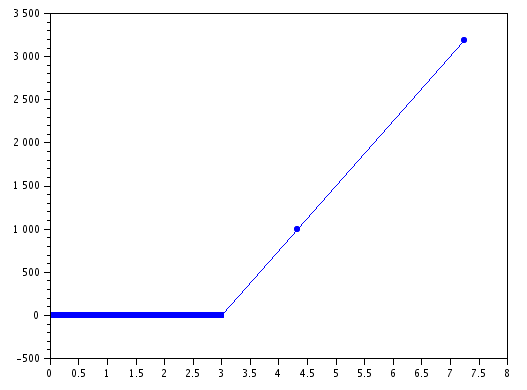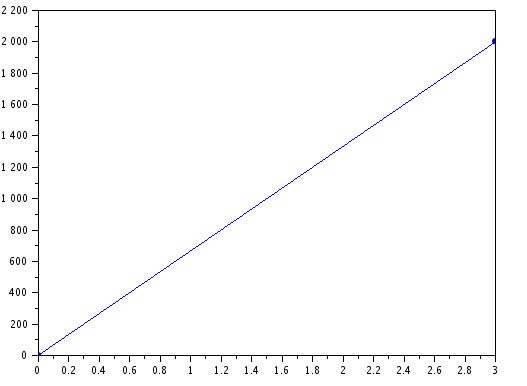Big time increase is because the ecuation solver is constantly oscilating around the 0 value of the queue (for short times it goes below to 0).

In total que QSS integrator produced 7984 K events. Below is a plot of the first 10 K events (right) and of events 5K to 15K (left).

To review: the plot on the left was expected because of the oscilations. But I still don't understand why the first 5000K events produce always the same exact value -0.0000010. I thought QSS only schedules an event when a significan delta is detected in the value (which is not the case here).
routerQueue.value(1:50)
ans =
column 1 to 6
0. 0.000001 8.000D-10 8.000D-10 - 0.0000010 - 0.0000010
column 7 to 12
- 0.0000010 - 0.0000010 - 0.0000010 - 0.0000010 - 0.0000010 - 0.0000010
column 13 to 18
- 0.0000010 - 0.0000010 - 0.0000010 - 0.0000010 - 0.0000010 - 0.0000010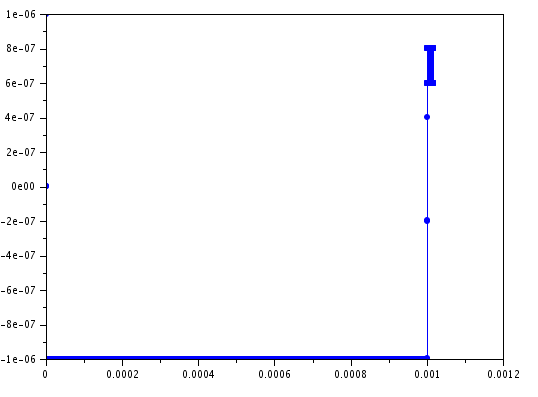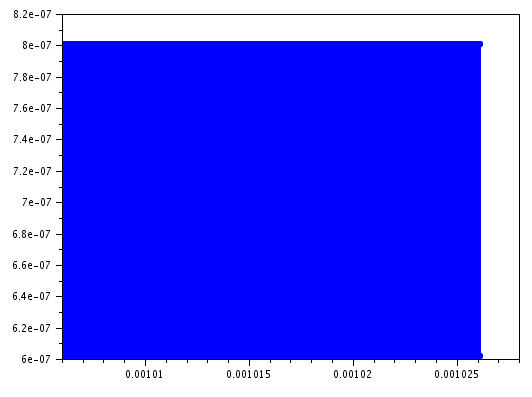One option no solve this problem is to reduce QSS delta. We are only interested in changes of the queueSize in the order of the unit (if it changes more than 1 packet in size).

Setting the following parameters in the QSS integrator yield similar results, but the execution finished in less than 1s (The integrator produced only 37 events.).

QSS Params
dqmin
= 1
dqrel = 0 (uniform)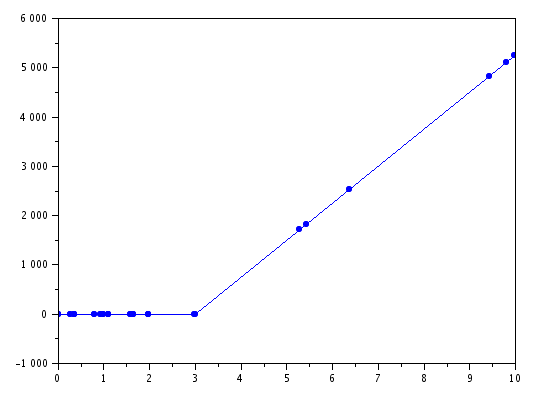One disadvante of this setting is the expected loss of precition. This is notizable for example looking at the minimum value of the queue, which is -1.
In order to stay on the safe side one could set dqrel =1e-3 (original value) to let the integrator dynamically manage the delta. Also, not to have big discrepancies of negative values one could set dqmin = 0.1. This these setting the following result is obteined, the simulation finishes in less than 1s, produces 103 events and min queueSize=-0.1.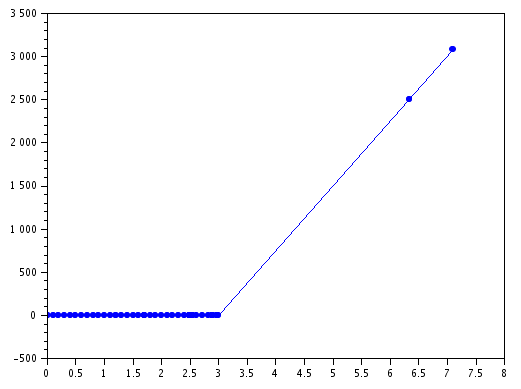In order to reduce the impact of the negative values in the queueSize calculation (which is unavoidable in the continous world), one could use a saturation model in all calculations that require the queueSize (for example the queueing_delay).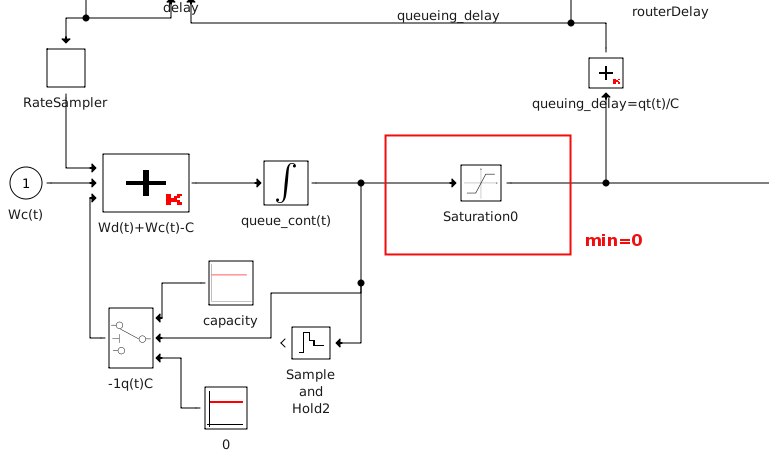### Idea

The previous dynamics describe a switch with infinite buffer capacity. If the buffer capacity is limited the switch discards packets when the threadhold is reached.

From the discrete packets point of view, if the next packet does not fit in the buffer then that packet is discarded. But this idea is hard to integrate with the continous flow as the buffer size in the continous world is not a constant number but rather a "rate". Below there is an idea on how to integrate them.

From the continuos perspective, if the queue reached its maxium capacity then the exceeding traffic is discarded. In terms of equations one could calculate the discard probability dp as follows:

dp(t) = 0 if q(t)<Buffer_max
dp(t) = W(t) - C otherwise

Once the queue reached its maximum capacity, its size should not continue growing. To take this into account, we modify the original q(t) to also substract the discard probability:### Implementation - Continous part

To implement the previous ecuations, we first added the pd(t) calculation. The "Wd+Wc-C" model adds those values to calculate the pd(t).
The "pd(t)" model is a switch, that uses the "Wd+Wc-C" value only when q(t)>buffer_max, otherwise it sends 0. The buffer_max parameter is configured in this switch model (level)
The output of "pd(t)" model is now used to as an extra term in the calculation of the queue size to implement the new q(t)/dt= -C + Wc(t) + Wd(t) - pd(t)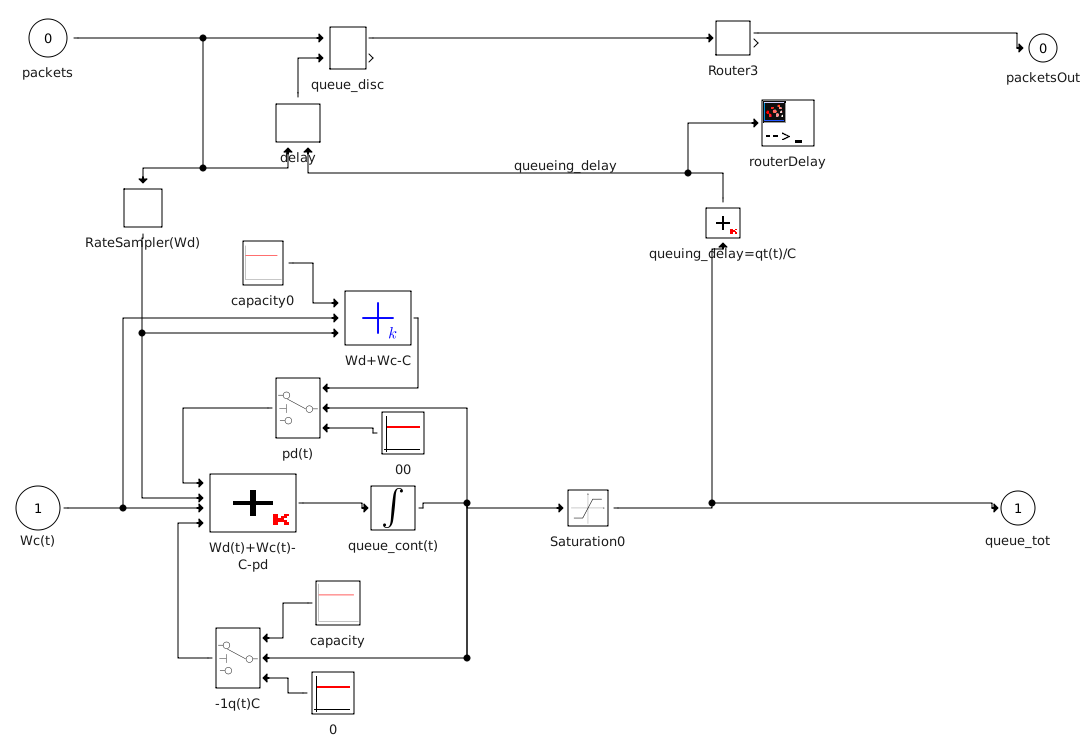To test the discard probability implementation, we set the buff_max=500 and Router_capacity=1250. Using the same pulse model to generate the Wc(t) (1 at the beggining, and at t=3 Wc=2000). The discrete part is set with Wd=1 (one packet per second).

It can be seen that the queue size is kept not bigger than the buffer_max. Also the discarProbabilty is set to 750=2000-1250 little after t=3 (as soon as the queue reaches its maxBuffer).
There are small spikes in the pd(t). These are the moments when discrete packets arrive, and perfectly discribe that in those moments more traffic should be discarded.

[Left=q(t) ; Right=pd(t) ]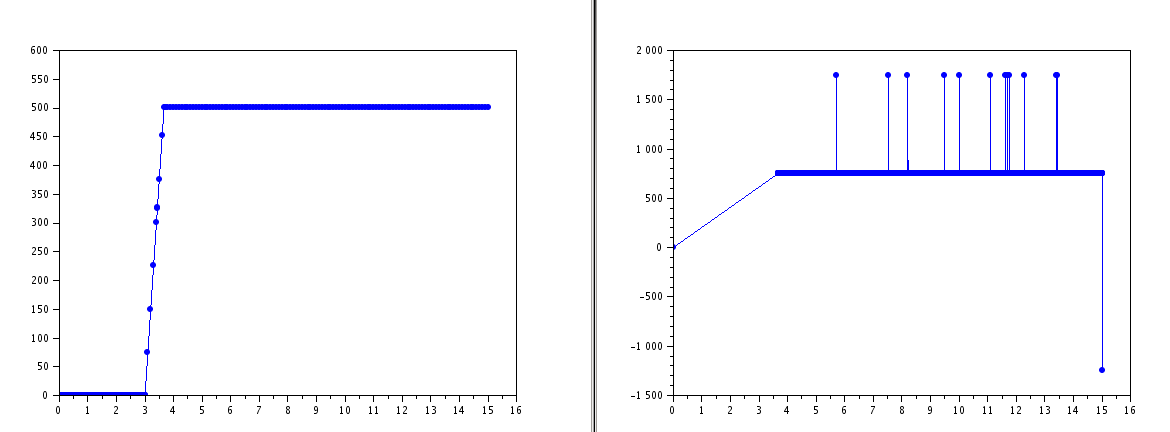Mixing with discrete traffic

Same experiment as before, but setting a higher Wd rate=300.
It can be seen that the queue perfectly adapts to both flows, never going higher than buff_max. Also the discar probability changes accordin to the discrete ups&downs.
The mean(Wd)=306.2 (it was configured to send 300 packets per second). The mean(pd)=1059.2428 (which matches Wd+Wc-C=300+2000-1250=1050)

[Left=q(t) ; Center=pd(t) ; Right=Wd ]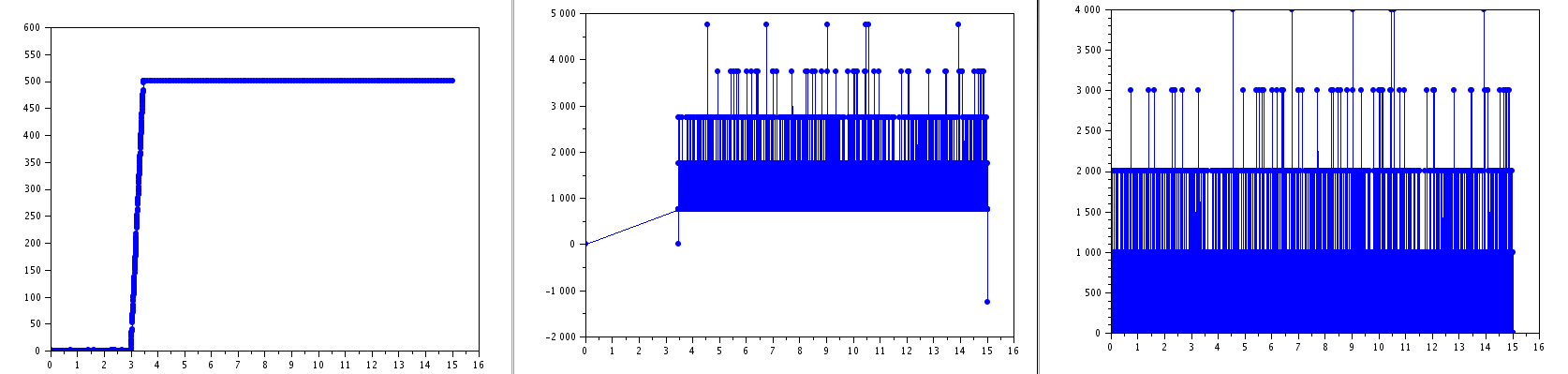Now the queue is accurately representing saturation and we can calculate the discard probability. But no packets are actually discarded. To implement this feature we will add a discretePacketDiscarder model.
One would be tempted to program the discretePacketDiscarder model so that it discards arriving packets when the queue is full. But this would result in an unfair discard of all discrete packets while the continous flow will still be sent at full BW. For example, if capacity=1000, Wc=1200, Wd=500, then 1200+500-1000=700 packets will be discarded in total. But the queue will be full at all times so all discrete packets will be discarded. Instead we would like to be equitative and discard discrete packets at the same rate which continuos flow is discarding. So, 700 discarded packets represent the 41% of total input, so we would like 500*0.41=205 discrete packets to be discarded.

So the discretePacketDiscarder model will discard packets at the rate given by#### Implementation

We added the packetDiscard model. This model receives all incomming packets. It also receives the calculation of the above equation: pd/(Wc+Wd). This models discards incomming packets at the rate given by the second input port (using STDEVS random generation numbers).

Additionaly, we added a Saturation model after the calculation of pd(t). This is because when the Wc suddenlt reduces and the queue is full, the pd(t) can become negative. This in turn causes the queue to stay full (pd is negative so it would add instead of substract from the queue size). Also, it does not make sense that the discarded packets to go below 0. This satudation represents the restriction that pd > 0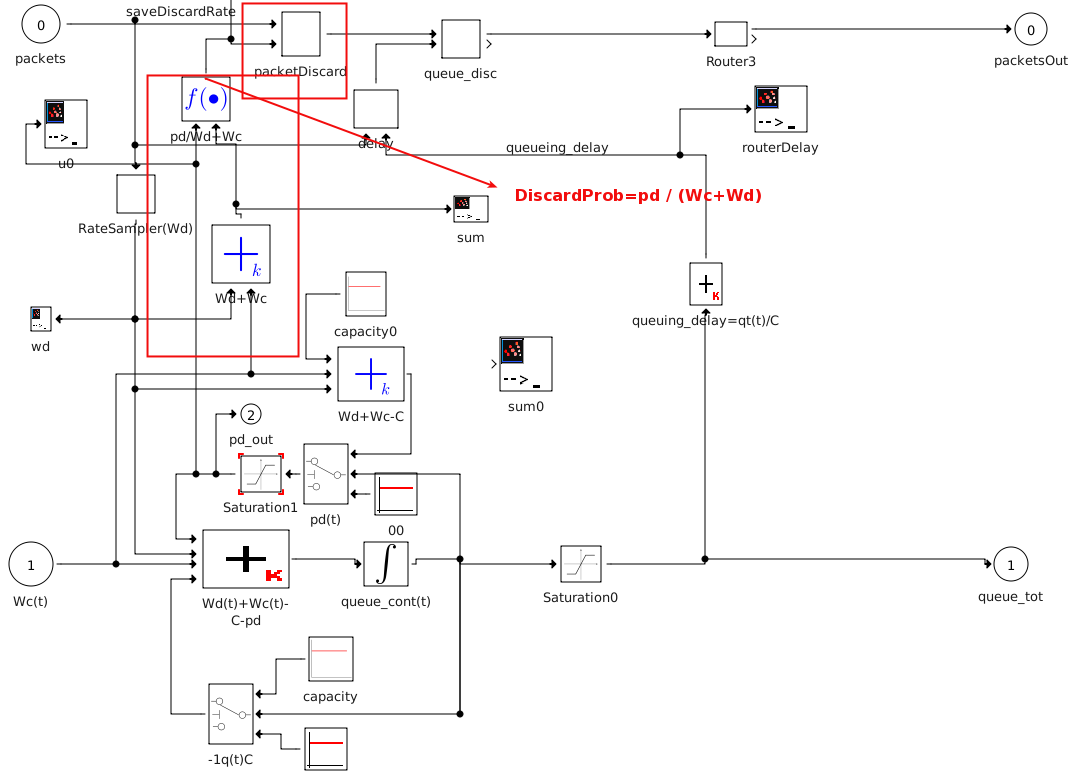#### Testing discards of discrete packets

configuration
We configured the model as follows: capacity=1000Hz, Wc=1200Hz, Wd=500Hz.
Then 1200+500-1000=700 packets will be discarded in total per second. So the router will discard in total 41% of the packets. If the router is fair between the discards of the fluid and discrete flows, it should discard 500*0.41=200 of the discrete packets per second.

results

It can be seen that as soon as the queue gets filled left) the rate at which discrete packets go out of the router dicreases because right) discards start apearing.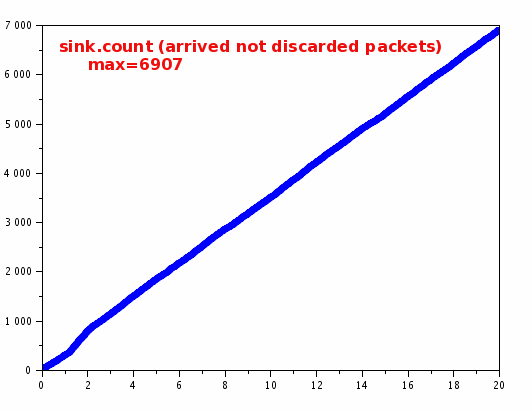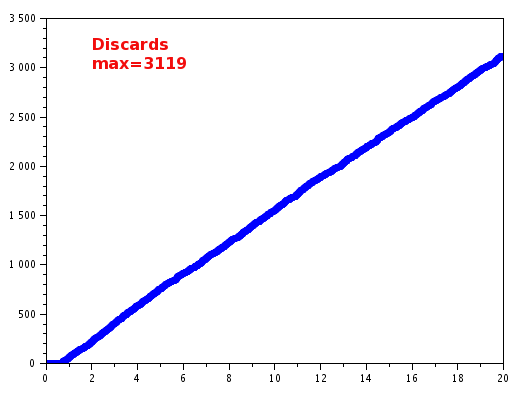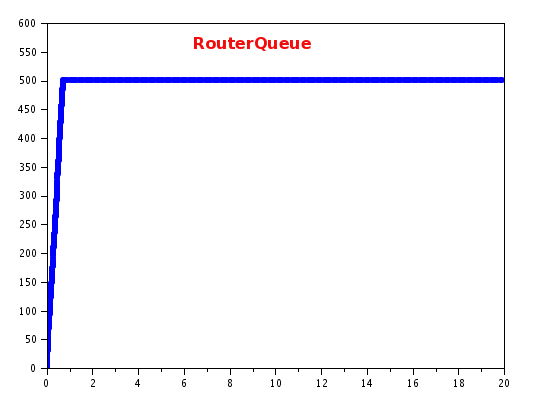Validation

Nevertheless, there were less discrete packets discarded than expected. We were expecting ~200 packets to be discarded per second and we had 3119/20=155.95. Indeed, because for the first 0.75s there are no discards it would be more fair to calculate 3119/19=164.157, still not quite all right. 3119 packets represent the ~31% of the total packets (we were expecting 41% to be discarded).

To understand this fault we explore some values from the simulation in this post ValidtionOfDiscreteDiscards.
We could not understand the root cause of the problem. We tracked the issue to a problem of miss match in the calculation of the DiscardProbability pd / (Wc +Wd) and the expected value. The mean E(DiscardProbability)  E[pd] / E[Wc+Wd]. This is the cause of the problem, but we can not understand why is it happening.
We found a possible solution increasing the samplingPeriod in the RateSampler model. Increasing the samplingPeriod generates less samples which intuitivelty should create less precision. But counter intuitively, it makes the discard packets to match what it is expected. The reason is still not understood completetly. Using a samplingPeriod of 0.1s (instead of the original 0.001s) makes the discarded packets percentage to be ~40.7% (the expected is 41%)
ValidtionOfDiscreteDiscards. has more details and tests.

Configuration 2

Now we test with a changing continous rate. I.e we use a Pulse model instead of a constant to represent the contious flow. The pulse will have amplitud=1200, InitialTime =3, FinalTime =15
We use the samplingPeriod=0.1s

results

generated flows: (left: discrete sampled) (right: continuos flow)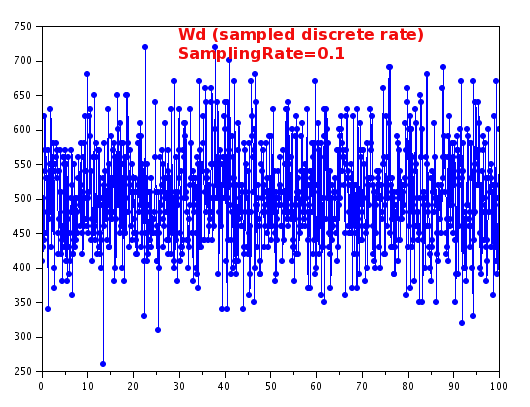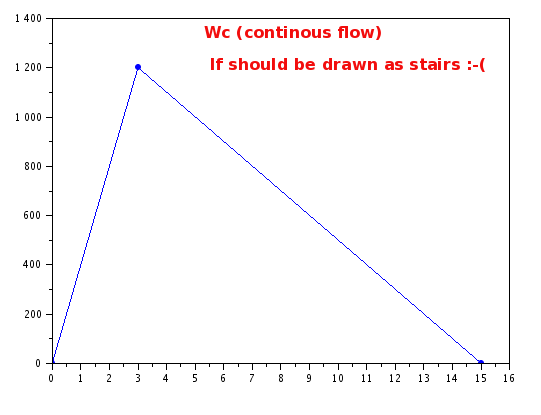QueueSize

It matches the expectation: it is empty at the begging and gets filled when the continous flow comes in.

Here we can see a drawback of this hybrid approach: the queueSize is calculated in a "continous manner". That is, it is calculated based on the incoming rates and outgoing capacity. The incoming rates are continous and thus they represent is some sense mean values. Because the discrete flow is transformed into a rate, the queue stays always in 0. This would not be the real case, where the queue will grow a little bit when bursts of packets arrive.
The accuracy can be managed setting the sampling rate. For the queue to grow, it requires that a sampledValue of the discreteFlow be higher than the capacity. Setting a very small sampling period will cause the sampledValue to be high every each time a packet arrives and cause queuing (but it will last for very little time). It is to say: this model is insensitive to bursts of discrete packets which occur in an interval smaller than the samplingPeriod.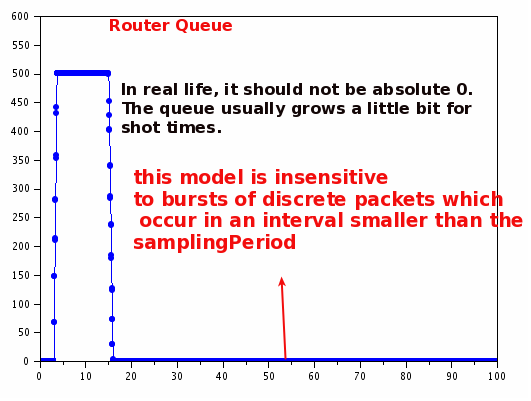Discard occur when the queue is full. When the Wc goes back to 0 discards disapear again.

There were a total of 2233 discrete packets discarded. The discards occur from t=3.75s to t=15s. During that period 11.25s*500packets=5625 packets were sent, so 2233 represent the 39.69% of the total packets. It was expected that ~40% of the packets to be discarded in that period.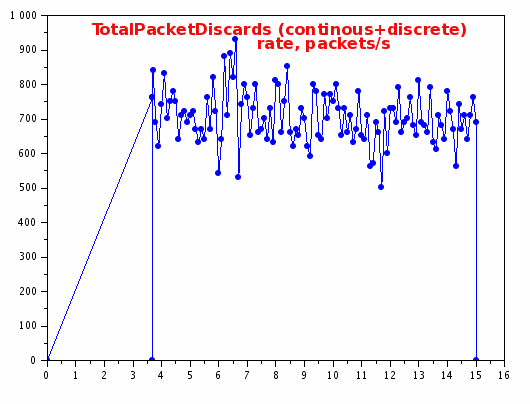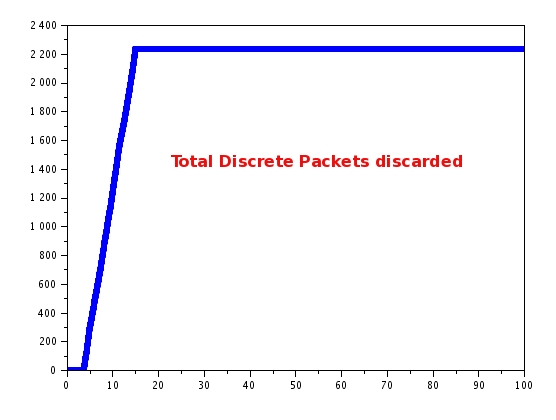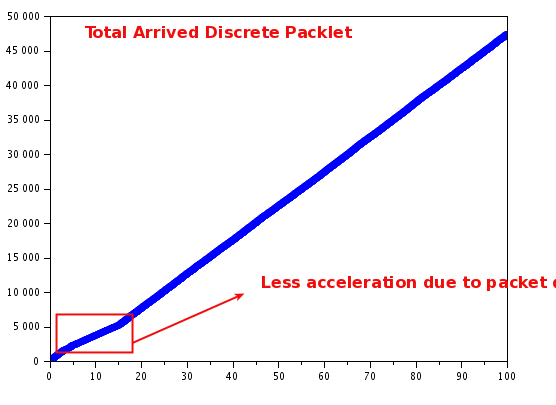-- MatiasAlejandroBonaventura - 2016-07-26

TWiki LatexModePlugin error messages:
Error! multiple equation labels 'eqn:one' defined. (Eqns. 1 and 2)
Error! multiple equation labels 'eqn:one' defined. (Eqns. 1 and 3)

Latex rendering error!! dvi file was not created.
Topic revision: r13 - 2016-08-26 - MatiasAlejandroBonaventuraLog In

Webs

Welcome GuestCern SearchTWiki SearchGoogle Search Main All websCopyright &© 2008-2019 by the contributing authors. All material on this collaboration platform is the property of the contributing authors.
Ideas, requests, problems regarding TWiki? Send feedback